Engineering ToolBox - Resources, Tools and Basic Information for Engineering and Design of Technical Applications!

# Beams Natural Vibration Frequency

## Estimate structures natural vibration frequency.

Vibrations in a long floor span and a lightweight construction may be an issue if the strength and stability of the structure and human sensitivity is compromised. Vibrations in structures are activated by dynamic periodic forces - like wind, people, traffic and rotating machinery.

There are in general no problems with vibrations for normal floors with span/dept ratio less than 25. For lightweight structures with span above 8 m (24 ft) vibrations may occur. In general - as a rule of thumb - the natural frequency of a structure should be greater than 4.5 Hz (1/s)

### Structures with Concentrated Mass

f = (1 / (2 π)) (g / δ)0.5                         (1)

where

f = natural frequency (Hz)

g = acceleration of gravity (9.81 m/s2)

Note! - static dead load for a structure is load due to it's own weight or the weight of mass that is fixed to the structure.

### Structures with Distributed Mass

General rule for most structures

f = a / (δ)0.5                         (2)

a = numerical factor (in general 18)

The numerical factor a can be calculated to 15.75 for a single lumped system but varies in general between 16 and 20 for similar systems. For practical solutions a factor of 18 is considered to give sufficient accuracy.

### Simply Supported Structure - Mass Concentrated in the Center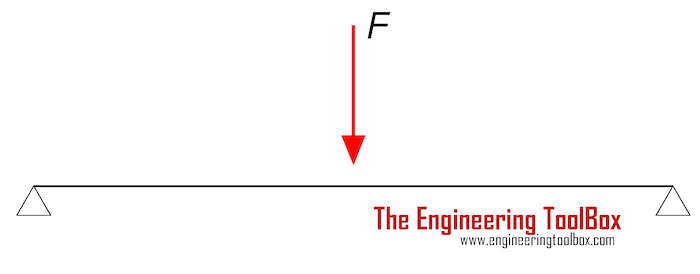For a simply supported structure with the mass - or load due to gravitational force weight - acting in the center, the natural frequency can be estimated as

f = (1 / (2 π)) (48 E I / M L3)0.5                            (3)

where

M = concentrated mass (kg)

### Simply Supported Structure - Sagging with Distributed Mass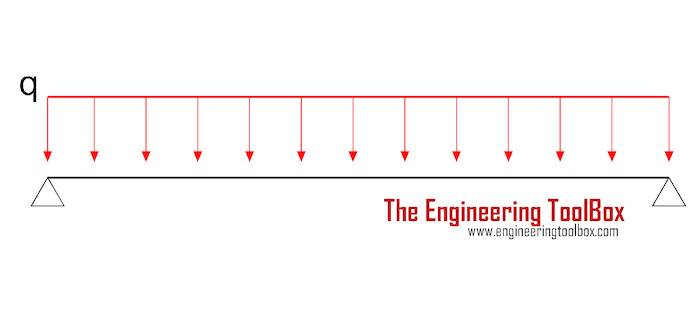For a simply sagging supported structure with distributed mass - or load due to gravitational force - can be estimated as

f = (π / 2) (E I / q L4)0.5                            (4)

#### Example - Natural Frequency of Beam

The natural frequency of an unloaded (only its own weight - dead load) 12 m long DIN 1025 I 200 steel beam  with Moment of Inertia 2140 cm4 (2140 10-8 m4) and Modulus of Elasticity 200 109 N/m2 and mass 26.2 kg/m can be calculated as

f = (π / 2) ((200 109 N/m2) (2140 10-8 m4) / (26.2 kg/m) (12 m)4)0.5

= 4.4 Hz  - vibrations are likely to occur

The natural frequency of the same beam shortened to 10 m can be calculated as

f = (π / 2) ((200 109 N/m2) (2140 10-8 m4) / (26.2 kg/m) (10 m)4)0.5

= 6.3 Hz  - vibrations are not likely to occur

### Simply Supported Structure - Contraflexure with Distributed Mass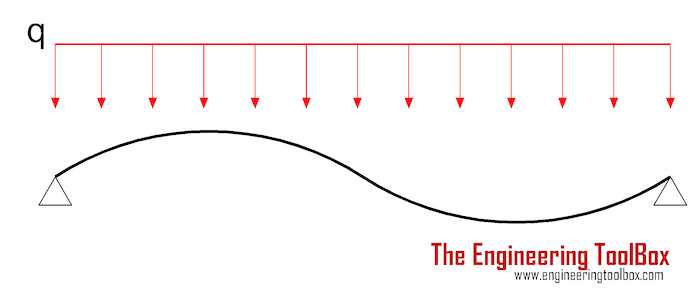For a simply contraflexure supported structure with distributed mass - or dead load due to gravitational force - can be estimated as

f = 2 π (E I / q L4)0.5                            (5)

### Cantilever with Mass Concentrated at the End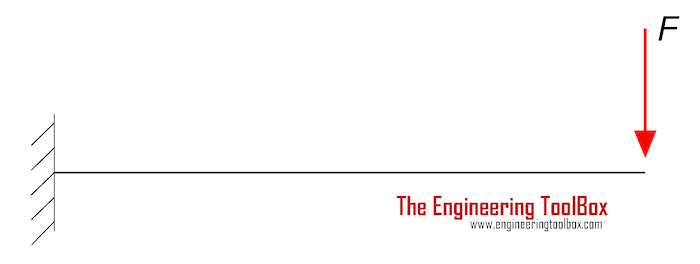For a cantilever structure with the mass - or dead load due to gravitational force - concentrated at the end, the natural frequency can be estimated as

f = (1 / (2 π)) (3 E I / F L3)0.5                            (6)

### Cantilever with Distributed Mass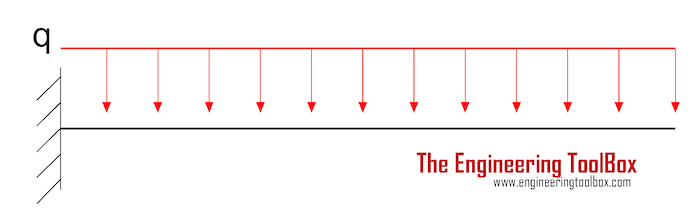For a cantilever structure with distributed mass - or dead load due to gravitational force - the natural frequency can be estimated as

f = 0.56 (E I / q L4)0.5                            (7)

### Structure with Fixed Ends and Distributed Mass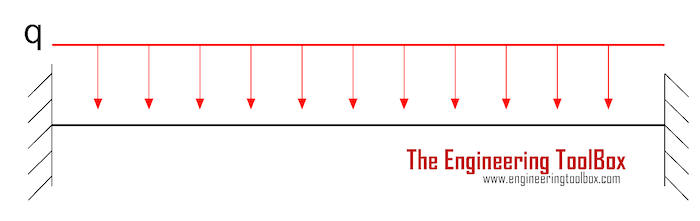For a structure with fixed ends and distributed mass - or dead load due to gravitational force - the natural frequency can be estimated as

f = 3.56 (E I / q L4)0.5                            (8)

## Related Topics

• Beams and Columns - Deflection and stress, moment of inertia, section modulus and technical information of beams and columns.

## Engineering ToolBox - SketchUp Extension - Online 3D modeling!

Add standard and customized parametric components - like flange beams, lumbers, piping, stairs and more - to your Sketchup model with the Engineering ToolBox - SketchUp Extension - enabled for use with the amazing, fun and free SketchUp Make and SketchUp Pro .Add the Engineering ToolBox extension to your SketchUp from the SketchUp Pro Sketchup Extension Warehouse!

Translate

## Privacy

We don't collect information from our users. Only emails and answers are saved in our archive. Cookies are only used in the browser to improve user experience.

Some of our calculators and applications let you save application data to your local computer. These applications will - due to browser restrictions - send data between your browser and our server. We don't save this data.

## Citation

• Engineering ToolBox, (2017). Beams Natural Vibration Frequency. [online] Available at: https://www.engineeringtoolbox.com/structures-vibration-frequency-d_1989.html [Accessed Day Mo. Year].

Modify access date.

. .

#### Scientific Online Calculator6 24